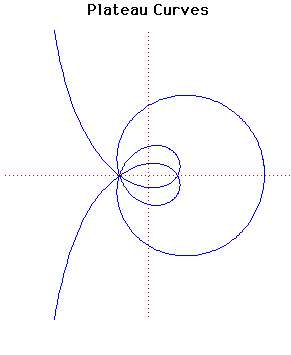# Plateau CurvesParametric Cartesian equation:
x = a sin(m + n)t/sin(m - n)t, y = 2a sin(mt)sin(nt)/sin(m - n)t

Click below to see one of the Associated curves.

Click THIS LINK to experiment interactively with this curve and its associated curves.

This curve was studied by the Belgium physicist and mathematician Joseph Plateau.

If m = 2n the Plateau curves become a circle, centre (1, 0) and radius 2.

The particular curves drawn above are with the parameters m = 5, n = 3.

JOC/EFR/BS January 1997